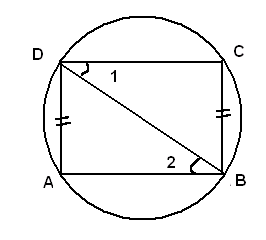# SSC CGL 2018 Practice Test Papers | Quantitative Aptitude (Day-3)

Dear Aspirants, Here we have given the Important SSC CGL Exam 2018 Practice Test Papers. Candidates those who are preparing for SSC CGL 2018 can practice these questions to get more confidence to Crack SSC CGL 2018 Examination.

[WpProQuiz 2638]

Click “Start Quiz” to attend these Questions and view Explanation

1. If a/b+b/a=1,a≠0, b≠0, the value of a3+b3 is ______

(a) 0

(b) 1

(c)  -1

(d) 2

1. ABCD is a cyclic parallelogram. The angle c is equal to____

(a) 30

(b) 60

(c) 90

(d) 45

1. If sinѲ+ cosecѲ= 2 then sin100Ѳ+ cosec100Ѳ is equal to

(a) 1

(b) 2

(c) 200

(d) 100

4 . A man walks a distance of 40 km from home to his office at 8 km/hr, he was late by 80 minutes.

If he walks at 10 km/hr He will reach the office____

(a) 20 minutes late

(b) 30 minutes late

(c) 30 minutes earlier

(d) 20 minutes earlier

1. If 2x+8=82x+1 then what is the value of x?

(a) 3

(b) 5

(c) 1

(d) 2

1. Fraction between 2/5 and 4/9 is

(a) 3/7

(b) 1/2

(c) 2/3

(d) 4/5

1. If 17200 is divided by 18, the remainder is______

(a) 17

(b) 1

(c) 16

(d) 2

8. Three persons A, B , C invest in a business in the ratio 8:5:7. After 3 months A left the business. If the total profit is 80000 what is difference of profit between B and C?

1. 12000
2. 20000
3. 30000
4. 15000

9). The least positive number when subtracted from 5 equals to 6 times the reciprocal of its number. What is the number?

a.6

b.2

c.3

d.10

­ 10). If cos4A-sin4A = X, then value of X is?

a.cos2A+1

b. cos2A -1

c.2cos2A+1

d. 2cos2A-1

11. Five bells A, B, C, D,E ring at intervals of 3,6,9,18 and 36 minutes and another bell F rings at an interval of X minutes. All the six bells ring together at every 180 minutes. How many X values are possible?

1. 10
2. 5
3. 4
4. 6

a/b+b/a=1

=>  (a2+b2)/ab=1

=> a2+b2=ab

=> a2+b2-ab=0

Therefore a3+b3=(a+b)(a2-ab+b2)=0ABCD is acyclic parallelogram.

∠A+∠C=180

2∠C=180

∠C=90

sinѲ+ cosecѲ= 2

sinѲ+1/ sinѲ=2

sin2 Ѳ-2 sinѲ+1=0

(sinѲ-1)2=0

sinѲ=1=> cosecѲ=1

sin100Ѳ+ cosec100Ѳ=1+1=2

Time taken to cover 40 km at the speed of 5 km/hr =40/8=5 hrs

Fixed time= 5 hrs  – 40 minutes= 4 hrs 20 minutes

Time taken to cover 40 km at the speed of 10 km/hr= 40/10=4 hrs

Required time= 4 hrs 20 minutes-4 hrs = 20 minutes earlier

2x+8=23( 2x+1)

X+8=3(2x+1)

X+8=6x+3

5x=5

X=1

2/5 = 0.40 and   4/9 =0.44

3/7=0.42

1/2=0.5

2/3=0.66

4/5=0.8

17200 = (18=1)200

17200/18 = (18=1)200/18

Remainder=1

8x*2: 5x*12: 7x*12= 4:15:21

80000/40x*(21-15)x=12000

(5-x)=6/x

5x-x2-6=0

x= 2,3

Least number is 2

cos4A− sin4A = (cos2A+sin 2 A) (cos 2A-sin 2A)

=1(cos2A-(1-cos2A))      ∴ (cos2x+sin 2x =1)

=2cos2A-1